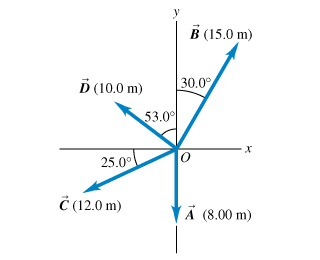# Problem: For the vectors A and B in the figure , use a scale drawing to find the magnitude of the vector sum A+B .Find the magnitude of the vector difference A - B.Use your answers to find the magnitude of -A - B.Find the magnitude of B - A.Find the direction of the vector sum A+B .Find the direction of the vector difference A - B.Find the direction of -A - B.Find the direction of B - A.

⚠️Our tutors found the solution shown to be helpful for the problem you're searching for. We don't have the exact solution yet.

###### Problem Details

For the vectors A and B in the figure, use a scale drawing to find the magnitude of the vector sum A+B .

Find the magnitude of the vector difference A - B.

Find the magnitude of B - A.

Find the direction of the vector sum A+B .

Find the direction of the vector difference A - B.

Find the direction of -A - B.

Find the direction of B - A.